# How to Create Formulas in Excel?

One of the most powerful features in Excel is the ability to calculate numerical information using formulas. Just like a calculator, Excel can add, subtract, multiply, and divide. This tutorial explains how to write formulas in Excel, beginning with very simple ones. We will learn how to create a formula in Excel using three ways,

1. Constants,
2. Cell references and
3. Defined names.

Also, we will see how to make formulas using the function wizard or enter a function directly in a cell.

Excel Vlookup formula – Guidebook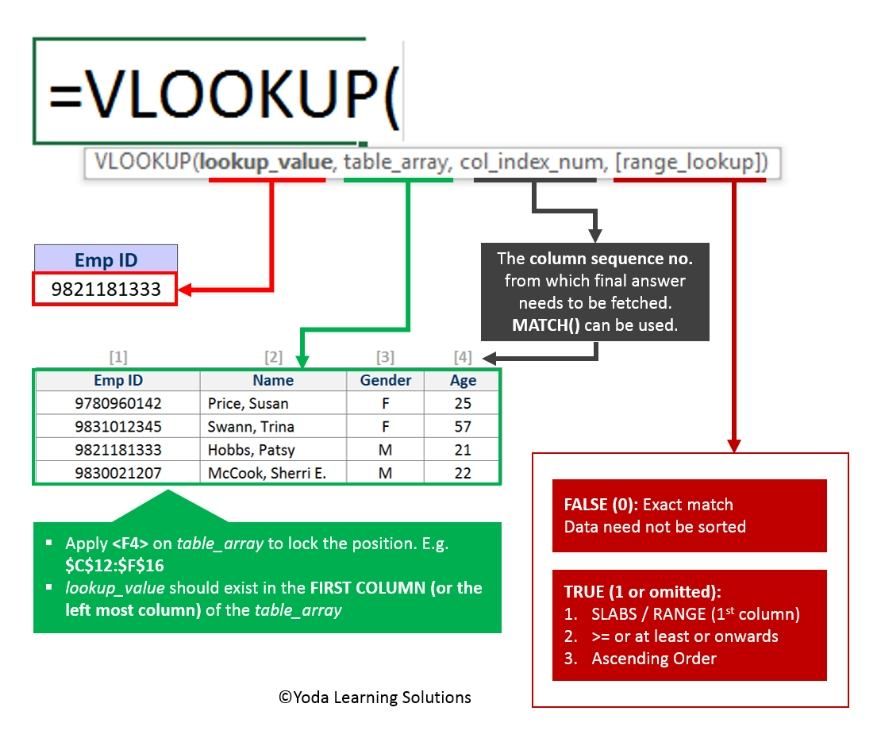If Yes, you will enjoy this guidebook on ‘Excel Vlookup Formulas’ – VLOOKUP, HLOOKUP, MATCH & INDEX.

Remember: For starters, any Excel formula begins with the equal sign (=). So, whatever formula you are going to write, begin by typing = either in the destination cell or in the Excel formula bar. Now, let’s have a closer look at how we can create formulas in Excel.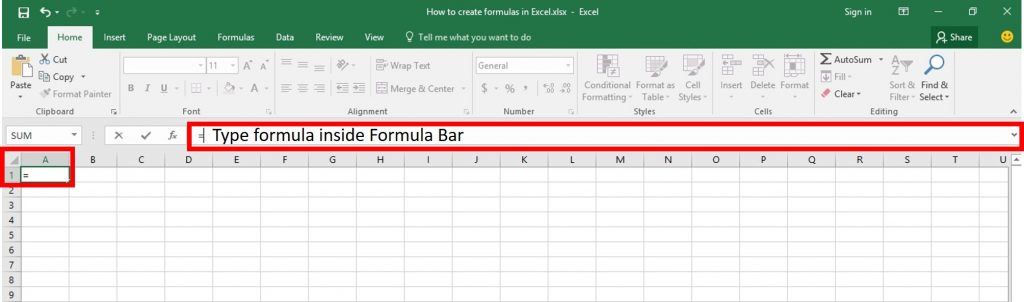## How to make a simple Excel formula by using constants and operators

In Microsoft Excel formulas, constants are numbers, dates or text values that you enter directly in a formula. To create a simple Excel formula using constants, let’s go through the following steps:

In this example, we have selected cell A1. Now Type the equal symbol (=).

Now we can start typing our formula here. In this stage, we can type the formula in 2 ways. One is typing the formula directly inside the cell and another way is to select the cell and type the formula in Formula Bar as like above picture.

Step 1: Select a Cell.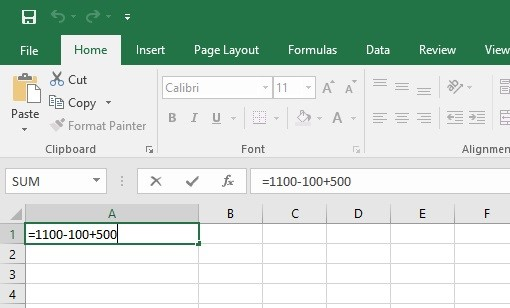Step 2: Type the equation you want to calculate.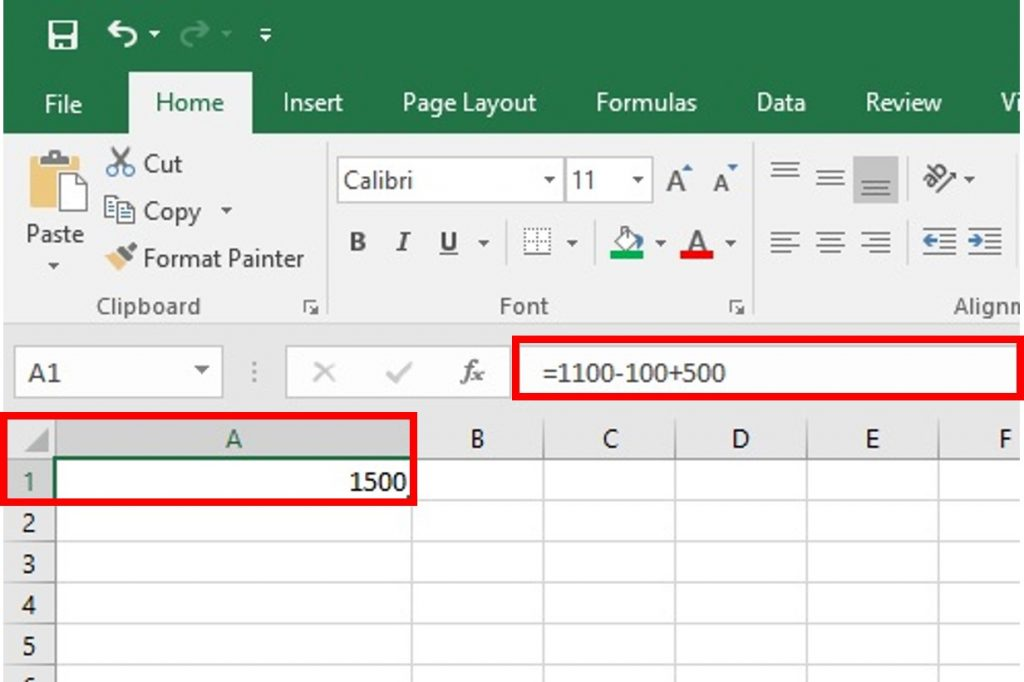Step 03: Press the Enter key to complete formula.

Still confuse about how to create formula is Excel? This tips will solved your querry

Tips 1: We can notice that in above picture when we select this cell to contain a formula, It shows the result 1500, but the formula bar is showing the formula inside this cell (=1100-100+500). But when we double-click on the cell, we can see the formula also inside the cell.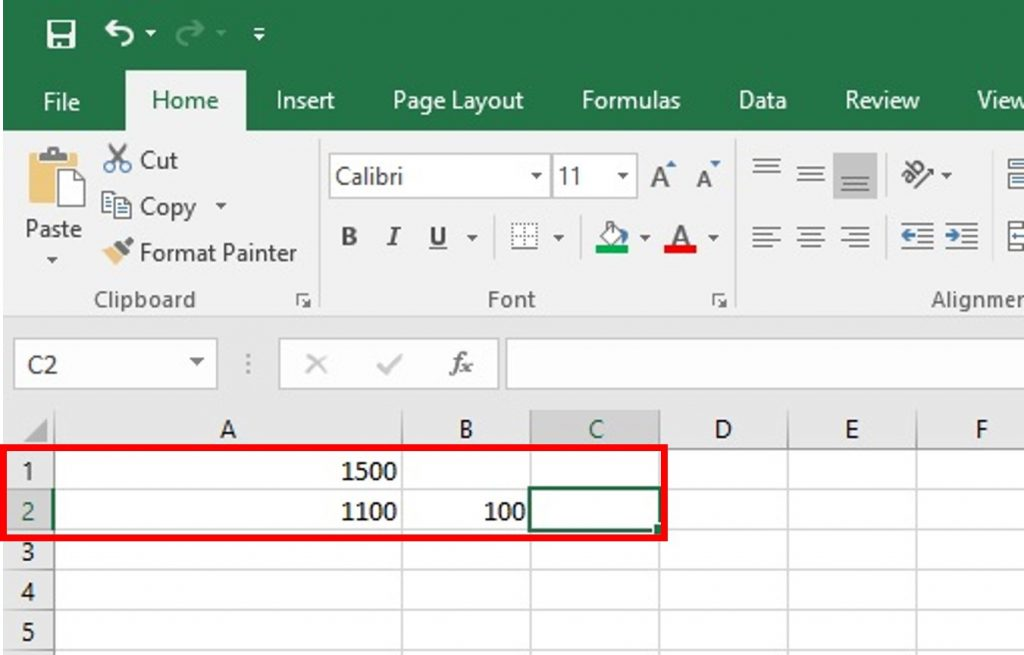In our next steps, we will see

Excel Vlookup formula – GuidebookIf Yes, you will enjoy this guidebook on ‘Excel Vlookup Formulas’ – VLOOKUP, HLOOKUP, MATCH & INDEX.

## How to write formulas in Excel using cell references?

Instead of entering values directly in our Excel formula, we can refer to the cells, containing those values.

For example, if you want to subtract a value in cell B2 from the value in cell A2, you write the following subtraction formula: =A2-B2

To understand this in more detail, let’s go through next steps:

Step 4: In our that Excel sheet, type 1100 in cell A2 and Type 100 in cell B2. Now We will use C2 to calculate 1100-100 using cell references.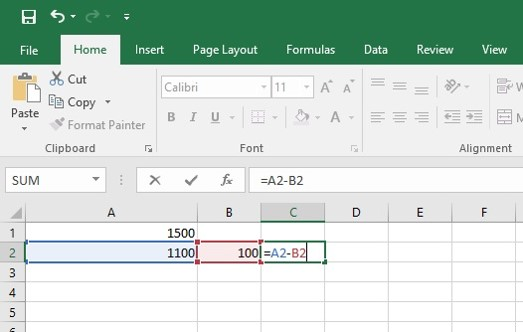Step 5: Type this formula in cell C2:

=A2-B2

In above picture, we can see that when we refer any cell in our formula, that cell is highlighting at this moment.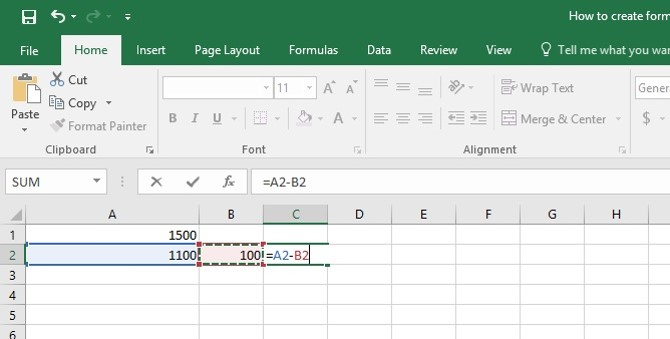Tips 2: When making such a formula, we can type the cell references directly in the formula, or click the cell and Excel will insert a corresponding cell reference in our formula. To add range reference, select the range of cells in the sheet. See the above picture.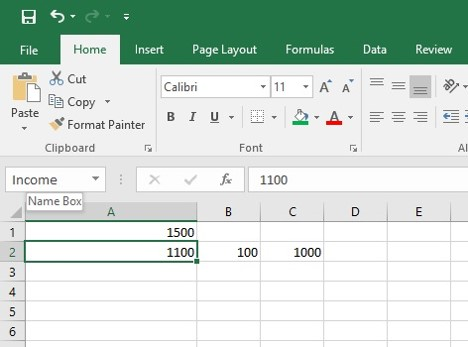## How to create an Excel formula by using defined names?!

We can create a name for a certain cell or a range of cells and then refer to that cell(s) in our Excel formulas by simply typing the name!

Step 6: In the upper side of sheet we can see Name Box. It contains the name of the cell. Click on cell A2. type Income in the Name Box.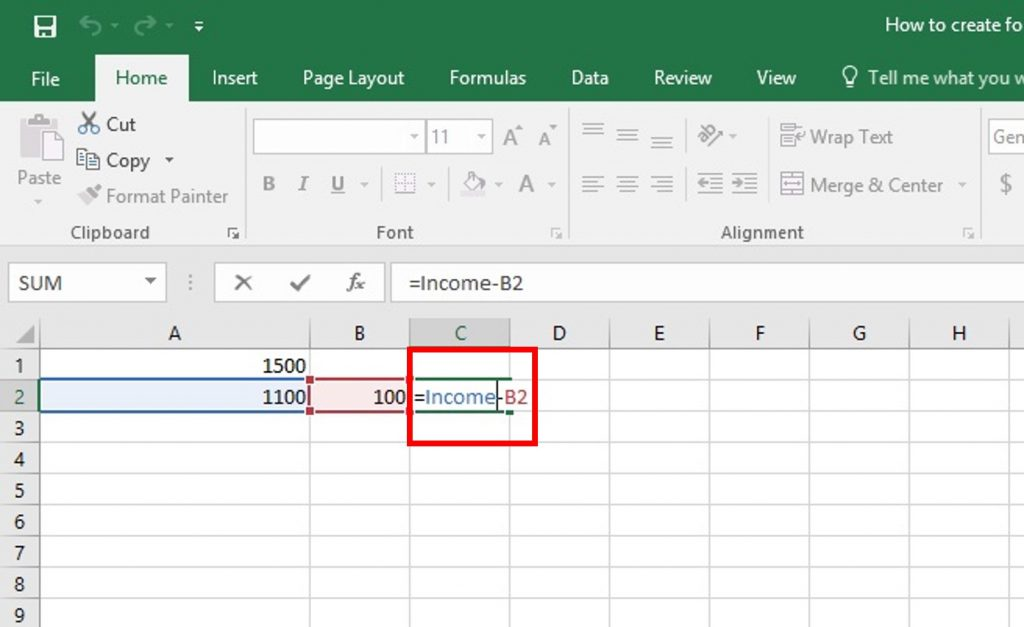Step 7: Now change our previous formula like this: =Income-B2

Now press ENTER. It is showing same result 1000.

So in this way, we can create an Excel formula by using Defined Names!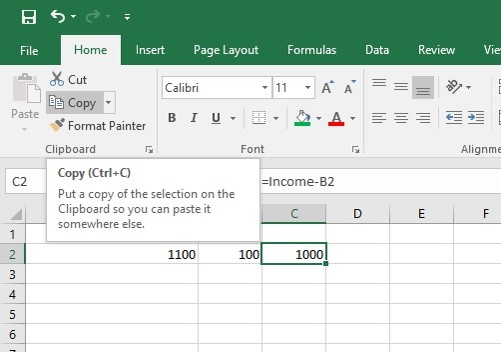Tips 3: Copy and Paste Values Without Formula

Normally if we Copy that cell C2 and Paste in another cell, it will not paste the value, but the formula. So how we can Copy and Paste Values Without Formulas?!

Step 8: Just copy normally the cell C2. Select the cell C2 and Select the “Home” tab, then Select “Copy” in the “Clipboard section. Or use shortcut command Ctrl+C from keyboard.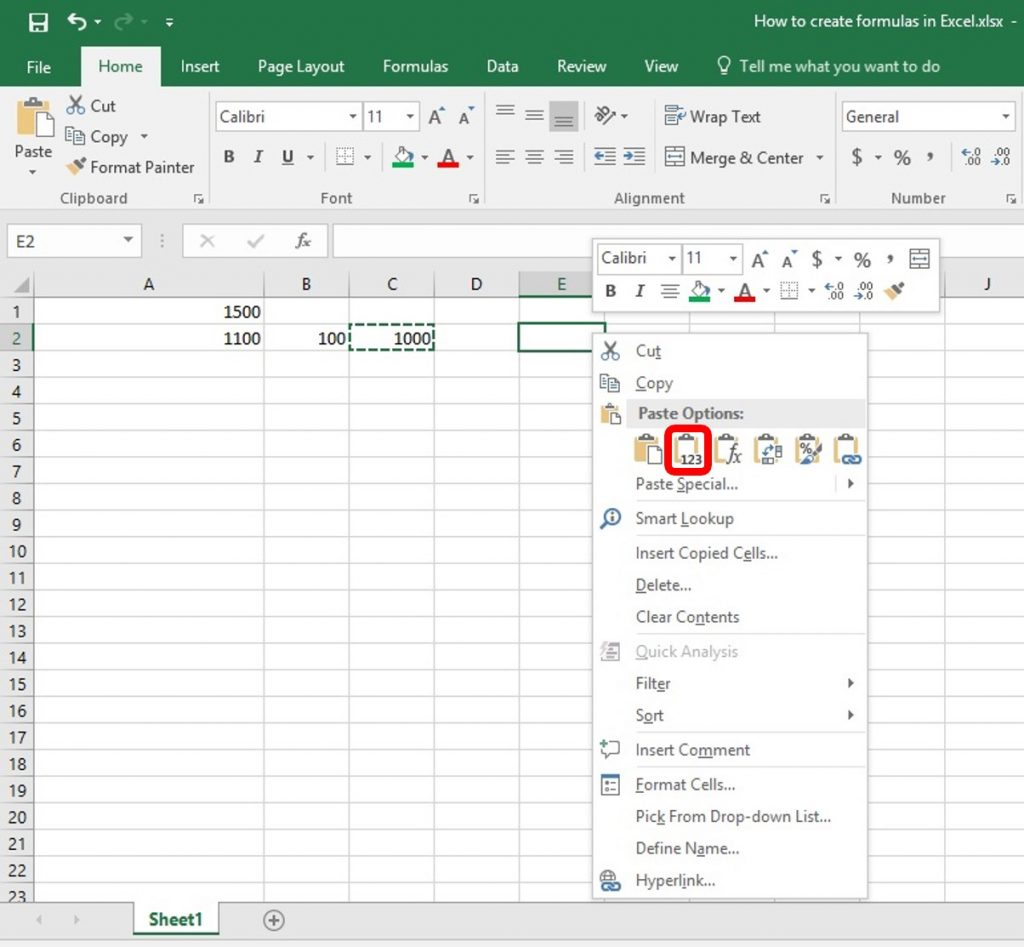Step 9: Right Click on Cell E2 then Select Value from the Paste Options Group. Now press ENTER. It will copy only the value, not a formula.

Now we have successfully learned How to create a formula and also how to copy value from the formula!

15 Pivot Tables Tricks for Pros

Most Popular Tricks are #3, #7 & #12